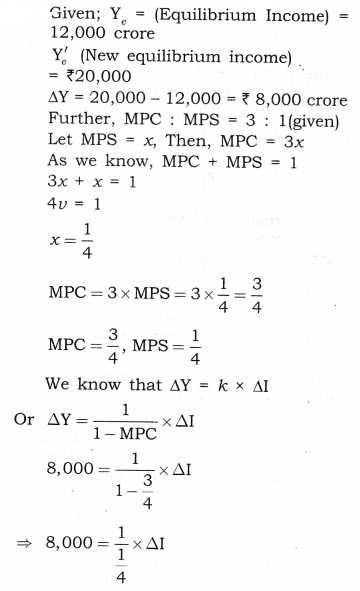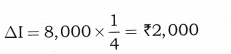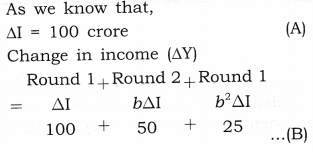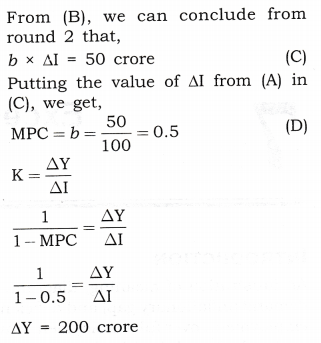# NCERT Solutions Class 12 Macro Economics Chapter-6 (National Income Determination and Multiplier)

NCERT Solutions Class 12 Macro Economics from class 12th Students will get the answers of Chapter-6 (National Income Determination and Multiplier) This chapter will help you to learn the basics and you should expect at least one question in your exam from this chapter.
We have given the answers of all the questions of NCERT Board Macro Economics Textbook in very easy language, which will be very easy for the students to understand and remember so that you can pass with good marks in your examination.

Class 12 Macro Economics

Chapter-6 (National Income Determination and Multiplier).

Questions and answers given in practice

Chapter-6 (National Income Determination and Multiplier)

NCERT TEXTBOOK QUESTIONS SOLVED

Question 1. Measure the level of ex-ante aggregate demand when autonomous investment and consumption expenditure (A) is Rs 50 crores, and MPS is 0.2 and level of income (Y) is Rs 4000 crores. State whether the economy is in equilibrium or not (cite reasons).[3-4 Marks]
Answer: As given in the examination problem, Equilibrium Income (Y) = Rs 4000 crore Autonomous Investment + Autonomous Consumption (A¯) = Rs 50 crore MPS = 0.2
So, MPC(b) = 1 – 0.2 = 0.8
(MPC = 1 – MPS)
AD = C¯ + bY + I = A + bY
= 50 + 0.8Y (A¯=C¯+I¯)
As we know, the equilibrium level of national income in two-sector model is determined where,
Y = 50 + 0.8Y
4000 = 50 + 0.8(4000)
4000 = 50 + 3200
4000 =3250
Hence, the economy is not in equilibrium.

Question 2. Explain ‘Paradox of Thrift’.[3-4 Marks]

1. The term thrift means savings and the paradox of thrift shows how an attempt by the economy as a whole to save more out of its current income will ultimately result in lower savings for the economy.
2.  If all the people in the economy make an effort to save more, then the total savings of the community will not increase, on the contrary they will decrease. This is called the paradox of thrift.
3.  Reasons for “Paradox of thrift” to operate:
(a) As we know that one person’s expenditure is another person’s income.
(b) If individual ‘A’ decides to save more by reducing his consumption expenditure, the income of individual ‘B’ will be less and individual ‘B’ in turn will spend less.
(c) Thus, if all individuals in the economy decide to save more, the income received by each individual will be less and overall income will fall and also lower will be the total savings.
4.  Diagram Representation: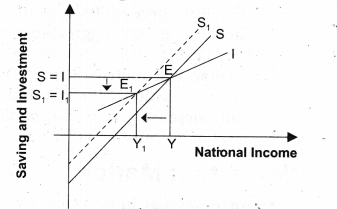In the above figure , we have induced investment function which makes the investment curve upward positively sloping. With the increase in savings, not only the equilibrium income falls, but also savings decline.

Question 3. What is Effective Demand? How will you derive the autonomous expenditure multiplier when price of final goods and the rate of interest are given?[6 Marks]

1. The level at which the economy is in equilibrium, i.e., where aggregate demand = aggregate supply, is called effective demand.
2. Under fixed price model, the value of planned (ex-ante) aggregate demand for final goods AD is equal to ex-ante consumption plus ex-ante investment expenditure.
AD = C + I = C¯ + bY + I¯
A¯ + bY
A¯ = 2 of all autonomous variable, i.e., (A¯=C¯+I¯))
3.  As we know that the equilibrium level of national income in two sector model is determined where,
Y=A¯+bY=A¯1b
4.  Diagrammatical representation,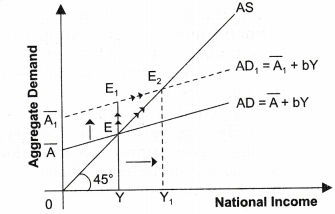In the above mentioned diagram, aggregate demand is measured on vertical axis and national income is measured on horizontal axis. Initially, at autonomous expenditure A¯, the equilibrium level of national income OY is determined at point E. But due to increase in autonomous expenditure from A¯ to A¯1, the aggregate demand curve shifts upward from AD to AD1 and at the same level of national income, i.e., OY, aggregate demand is greater than aggregate supply. Production will have to be increase to meet the excess demand. Consequently, national income will increase from OY to OY1 As, we know positive relationship exists between national income and consumption, so consumption will increase which will, increase the new aggregate demand AD1 , till we reach the new equilibrium level of output i.e., OY1 at E2

MORE QUESTIONS SOLVED

I.Very Short Answer Type Questions (1 Mark)
Question 1. If planned savings are greater than planned investments, what will be its effect on inventories? [CBSB Sample Paper 2008]

Question 2. What is meant by effective demand?
AnswerThe level at which the economy is in equilibrium, i.e., where aggregate demand = aggregate supply, is called effective demand.

Question 3. Define the term ‘multiplier’. How do we measure it?
AnswerThe ratio of change in national income (ΔY) due to change in investment (ΔI) is known as multiplier (K).
(K)=ΔYΔI

Question 4. An increase of Rs 1000 crore in invest¬ment leads to a rise of Rs 5000 crore in the national income. Calculate the value of multiplier.
=50001000 =Rs 5

Question 5. If investment multiplier is 1, what will be the value of marginal propensity to consume?
AnswerMarginal propensity to consume = 0.

Question 6. If marginal propensity to save is 0.1, calculate the value of multiplier.[CBSE 2003]

Question 7. If the value of marginal propensity to consume is 0.6, calculate the value of multiplier.
=11MPC=10.6=2.5

II.Multiple Choice Questions (1 Mark)
Question 1. If in an economy investment is greater than saving, national income of the economy,
(a) increases
(b) decreases
(c) remains constant
(d) None of them

Question 2. What happens to the level of national income, when aggregate supply falls short of aggregate demand?
(a) Increases (b) Decreases
(c) Constant (d) None of them

Question 3. What happens to the level of national income, when aggregate supply exceeds aggregate demand?
(a) Increases
(b) Decreases
(c) Remains constant
(d) None of them

Question 4. If MPC and MPS are equal, value of multiplier is,
(a) 2 (b) 1
(c) 5 (d) 3

Question 5. What is the relationship between MPS and Multiplier?
(a) Positive (b) Negative
(c) Constant (d) None of them

Question 6. What can be the minimum value of investment multiplier?
(a) 0 (b) 1
(c) 2 (d) 5

Question 7. What can be the maximum value of investment multiplier?
(a) 5 (b)
(c) 4 (d) 2

Question 8. If marginal propensity to save is 0.1 and increase in national income is Rs 500 crore, calculate increase in investment.
(a) 50 cr (b) 40 cr
(c) 10 cr (d) 2 cr

Question 9. The value of multiplier is:[CBSE Delhi 2015]
(a) 1MPC (b) 1MPS
(c) 11MPS (d)1MPC1

Question 10.  If MPC = 1, the value of multiplier is: [A/2015]
(a) 0 (b) 1
(c) Between 0 and 1 (d) Infinity

III. Short Answer Type Questions (3-4 Marks)
Question 1. What happens to the level of national income when aggregate demand falls short of aggregate supply?

1. As we know that equilibrium level of national income is determined under the given condition of aggregate demand and aggregate supply, and has tendency to stick to that level i.e., where aggregate demand is equal to aggregate supply.
2. As is given in the examination problem that when aggregate demand falls short of aggregate supply, then national income will decrease as shown in the above mentioned diagram.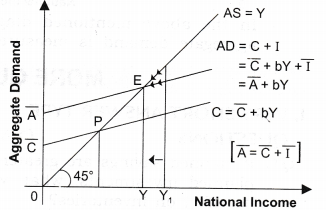3. When AD < AS [At Y1], then there would be stockpiling and producers will produce less. National income will fall and as we know positive relationship exists between national income and consumption, so consumption will fall, which will thereby decrease the aggregate demand till we reach the equilibrium.

Question 2. What happens to the level of national income when aggregate supply falls short of aggregate demand?

1.  As we know that equilibrium level of national income is determined under the given condition of aggregate demand and aggregate supply, and has tendency to stick to that level i.e., where aggregate demand is equal to aggregate supply.
2.  As is given in the examination problem that when aggregate supply falls short of aggregate demand, then national income will increase as shown in the given diagram: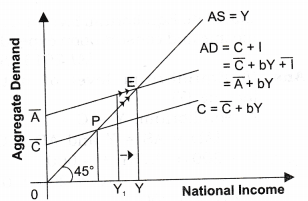3.  When AD > AS [At Y1], then production will have to be increased to meet the excess demand. Consequently, national income will increase. As we know that positive relationship exists between national income and consumption. So consumption will increase, which will thereby increase the aggregate demand till we reach the equilibrium.

Question 3. In an economy planned saving is greater than planned investment. Explain how the economy achieves equilibrium level of national income.[CBSE Sample Paper 2014, AI 04, CBSE 04, 05, 09]

1. It refers to the point that has come to be established under the given condition of aggregate demand and aggregate supply, and has tendency to stick to that level under this given condition. Therefore, AD = AS C + I = C + S I = C + S- C = S
2. As given in the examination problem, when planned saving is greater than planned investment, then national income will decrease as shown in the diagram.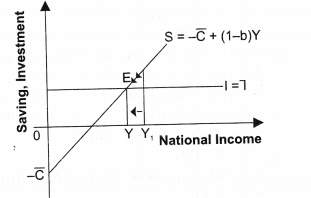3.  When saving > investment [at Y1], then there would be stockpiling and producers will produce less. National income will fall and as we know positive relationship exists between national income and saving, then saving will start falling until it becomes equal to investment. It is here the equilibrium level of income is derived.

Question 4. If planned saving is less than planned investment, what changes will bring economy in equilibrium?

1.  It refers to the point that has come to be established under the given condition of aggregate demand and aggregate supply, and has tendency to stick to that level under this given condition. Therefore,
AD = AS C + I = C + S I=C+S-C=S
2.  As given in the examination problem, when planned saving is less than planned investment, then national income will decrease as shown in the below diagram.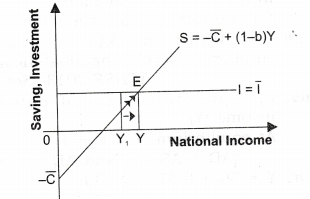3.  When, investment > saving [at Y1], then production will have to be increased to meet the excess demand. Consequently, national income will increase leading to rise in saving until saving becomes equal to investment. It is here that equilibrium level of income is established because what the savers intend to save becomes equal to what the investors intend to invest.

Question 5. Discuss relationship between MPC and multiplier. [AI 2006]

1.  If we put maximum value of MPC, i.e., 1, we get maximum value of multiplier, i.e., .
2. As against it, if we put minimum value of MPC, i.e., 0, we get the minimum value of multiplier, i.e. 1.
3. So, positive relationship exists between MPC and multiplier. It means when MPC increases, the multiplier also increases and vice-versa.MPCK

Question 6. Discuss relationship between MPS and multiplier. [CBSB 1994C, AI 1997]

1. If we put minimum of MPS i.e. 0, we get maximum value of multiplier, i.e., .
2. As against it if we get maximum value of MPS, i.e., 1, if we get minimum value of multiplier, i.e.,1.
3. Hence, inverse relationship exists between MPS and multiplier.
It means if MPS increases, the multiplier decreases and vice-versa.
MPSK

Question 7. c = 50 + 0.5Y is the consumption function; where C is consumption expenditure and Y is national income and investment expenditure is 72000 in an economy. Calculate:
(i) Equilibrium level of national income.
(ii) Consumption expenditure at equilibrium level of national income.[CBSE 2013 (Set III)]

1. Equilibrium level of national income (Y)
At equilibrium, Y = C + I
Or, Y = 50 + 0.5Y + 2,000
[C = 50 + 0.5Y]
0.5Y = 2,050
Y = Rs 4,100
2. Consumption expenditure at equilibrium level of national income.
Putting value of national income of 4,100 in consumption function, we get: C = 50 + 0.5 x 4,100 C = Rs 2,100
(a) Equilibrium level of national income = 74,100.
(b) Consumption expenditure at equilibrium level of national income = Rs 1740

Question 8. In an economy, C = 200 + 0.75 Y is the consumption function, where C is consumption expenditure and Y is national income and investment expenditure is Rs 4000 in an economy.Calculate Equilibrium level of national income and consumption expenditure.[AI 2013 (Set II)]

1. Equilibrium level of national income (Y)
At equilibrium, Y = C + I
[AD = AS = Y and AD = C + I] Or, Y = 200 + 0.75 Y + 4,000
[C = 200 + 0.75 Y]
0.25 Y = 1,200
Y = Rs 16,800
2.  Consumption expenditure at equilibrium level of national income.
Putting value of national income of 16,800 in consumption function, we get;
C = 200 + 0.75 x 16,800
C = Rs 12,800
(a) Equilibrium level of national income = Rs 16,800;
(b) Consumption expenditure at equilibrium level of national income = Rs 12,800

Question 9. From the following data about an economy, calculate (a) equilibrium level of national income and (b) total consumption expenditure at equilibrium level of national income.
(i) C = 200 + 0.5 Y is the consumption function, where C is consumption expenditure and Y is national income.
(ii) Investment expenditure is 1500.[AI 2013 (Set III)]

1.  Equilibrium level of national income (Y)
At equilibrium, Y = C + I
Or, Y = 200 + 0.5 Y + 1500
[C = 200 + 0.5 Y]
0.5 Y = 1,700
Y = Rs 3400
2. Consumption expenditure at equilibrium level of national income. Putting value of national income of 3400 in consumption function, we get; C = 200 + 0.5 x 3400 C = Rs 1900
(a) Equilibrium level of national income = Rs 3400.
(b) Consumption expenditure at equilibrium level of national income = Rs 1900

Question 10. Calculate equilibrium national income from the following data:
[AI 2013, C (Set I)] (i) Consumption expenditure at zero income = Rs 60
(ii) Marginal propensity to consume = 0.9 (iii) Investment = Rs 100

Answer: Equilibrium level of national income (Y) At Equilibrium, Y = C + I
Y =C¯ + b Y + I
Or, Y = 60 + 0.9 Y + 100
[C = 60 + 0.9 Y]
0.1 Y = 160
Y = Rs 1600

Question 11. Given consumption function C = 100 + 0.75 Y (where C = -consumption expenditure and Y = national income) and investment expenditure Rs 1,000, calculate: (i) Equilibrium level of national income; (ii) Consumption expenditure at equilibrium level of national income. [CBSE 2009]

1.  Equilibrium level of national income (Y)
At equilibrium, Y = C + I
[AD = AS = Y and AD = C + I] Or, Y = 100 + 0.75 Y + 1,000
[C = 100 + 0.75 Y]
0.25 Y = 1,100
Y = Rs 4,400
2. Consumption expenditure at equilibrium level of national income. Putting value of national income of 4,400 in consumption function, we get; C = 100 + 0.75 x 4,400 C = Rs 3,400
(a) Equilibrium level of national income = Rs 4,400;
(b) Consumption expenditure at equilibrium level of national income = Rs 3,400

Question 12. In an economy the consumption function is C = 500 + 0.75 Y, where C is consumption expenditure and Y is income. Calculate the equilibrium level of income and consumption expenditure when investment expenditure is Rs 5,000.[CBSE Foreign 2010 (I)]

1. Equilibrium level of national income (Y)
At equilibrium, Y = C + I
[AD = AS = Y and AD = C + I] Or, Y = 500 + 0.75 Y + 5,000
[C = 500 + 0.75 Y]
0.25 Y = 5,500
Y = Rs 22,000
2. Consumption expenditure at equilibrium level of national income. Putting value of National Income of 22,000 in consumption function, we get;
C = 500 + 0.75 x 22,000 C = Rs 17,000
Equilibrium level of income = Rs 22,000; Consumption expenditure at equilibrium level of income = Rs 17,000.

Question 13. Suppose that consumption equals C = 100 + 0.75 Y, and investment equals I = Rs 50 and Y = C + I. Find;
(i) The equilibrium level of income
(ii) The level of consumption at equilibrium, and
(iii) The level of saving at equilibrium.

1.  Y = C + I
Y = 100 + 0.75 Y + 50
Y – 0.75 Y = 150
0.25
2. C = 100 + 0.75 600 = Rs 550
3. S = Y – C = 600 – 550 = Rs 50

Question 14. Find national income from the following data: [CBSE 2012]Autonomous consumption = Rs 100 Marginal propensity to consume = 0.80 Investment = Rs 50
AnswerAs given in the examination problem, Autonomous consumption or C =150 Autonomous investment or I = Rs 50 MPC or b = 0.80 So, AD = C + I = C + bY + I = 100 + 0.8 Y + 50 = 150 + 0.8 Y
As we know that the equilibrium level of national income in two sector model is determined where,
Y = 150 + 0.8Y
Y – 0.8Y = 150 Y(1—0.8) = 150
Y=15020 =Rs 750

Question 15. An economy is in equilibrium. Find Marginal Propensity to Consume from the following: [CBSE 2015] National Income = 2000 Autonomous Consumption = 400 Investment Expenditure = 200
Answer: Autonomous consumption or C = Rs 400 Equilibrium level of income or Y = Rs 2000 MPC or b = ?
At equilibrium, Y = C + I
[AD = AS = Y and AD = C + I] Y = C + bY + I
2000 = 400 + b(2000) + 200 2000 – 600 = b(2000)
1400 = b(2000)
MPC(b) = 0.7

Question 16. An economy is in equilibrium. Calculate the Investment Expenditure from the following: [AI 2015] National Income = 800
Marginal Propensity to Save = 0.3 Autonomous Consumption =100

Answer: Autonomous consumption or C = Rs 100 Equilibrium level of income or Y = Rs 800 MPS = 0.3
MPC or b = 1 – MPS = 0.7 At equilibrium, Y = C + I
Y = 100 + 0.7Y + I
800 = 100 + 0.7(800) + I
800 = 100 + 560 + 1
I = 140

Question 17. As a result of increase in investment by Rs 125 crore national income increases by Rs 500 crore. Calculate marginal propensity to consume.[CBSE 2008]
We also know that Multiplier (k)
=11MPCor4=11MPC
or or 4 — 4 MPC = 1 or MPC = 3/4 = 0.75

Question 18. As a result of increase in investment national income increases by Rs 600 crore. If marginal propensity to consume is 0.75, calculate the increase in investment. [CBSE 2008]
=11MPC=110.75=10.25=4
We also know that k
=ChangeinIncome(ΔY)ChangeinInvestment(ΔI)
4=600ChangeinInvestment(ΔI)
Hence, change in investment(ΔI)
=Rs 150 crore
Increase in investment = Rs 150 crore

Question 19. If marginal propensity to consume is 0.9, what is the value of multiplier? How much investment is needed if national income increases by Rs 5,000 crore? [CBSE 2008]
=11MPC=110.9=10.1=10
We also know that k
=ChangeinIncome(ΔY)ChangeinInvestment(ΔI)
10=k=5000ChangeinInvestment(ΔI)
Hence, change in investment (ΔI)
= Rs 500 crores
Multiplier (k) = 10;
Increase in investment = Rs 500 crore

Question 20. An increase of Rs 250 crore in investment in an economy resulted in total increase in income of Rs 1,000 crore. Calculate the following: (a) Marginal propensity to consume (MPC), (b) Change in saving, (c) Change in consumption expenditure, (d) Value of multiplier. [CBSE Sample Paper 2008]
=ChangeinIncome(ΔY)ChangeinInvestment(ΔI)
=1000250=4
We know that Multiplier (k)
=11MPCor4=1MPC1
4-4MPC=1
MPC=3/4=0.75
MPC=ChangeinConsumption(ΔC)ChangeinIncome(ΔY)
0.75= ChangeinConsumption(ΔC)
So, change in consumption expen¬diture (C) = Rs 750 crore Change in Saving = Change in Income – Change in Consumption Expenditure So, Change in Saving = Rs 1,000 crore – Rs 750 crore = Rs 250 crore
(a) Marginal propensity to consume = 0.75
(b) Change in saving = Rs 250 crore
(c) Change in consumption expenditure = Rs 750 crore
(d) Value of multiplier = 4

IV.True Or False
Giving reasons, state whether the following statements are true or false:
Question 1. Value of investment multiplier varies between zero and infinity.[CBSE 2010]
Answer: False. The value of investment multiplier varies between unity and infinity. This can be proved as follows, When MPC = 0,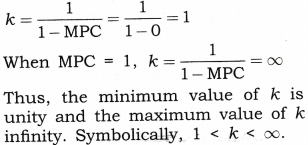Question 2. When marginal propensity to consume is zero, the value of investment multiplier will also be zero. [CBSE AI2010]
Answer: False. When MPC = 0, the value of investment multiplier will be equal to unity.
This is shown as,
Multiplier (k) =
When MPC = 0,k=1/(1-0)=1

Question 3. When marginal propensity to consume is greater than marginal propensity to save, the value of investment multiplier would be greater than 5. [CBSE 2010]
Answer False. Case I: When MPC > MPS (i.e., MPC = 0.6 and MPS = 0.4), the value of the investment multiplier,
k=1MPS=10.4=2.5
Case II: When MPC > MPS (i.e., MPC = 0.9 and MPS = 0.1), the value of the investment multiplier,
k=1MPS=10.1=10
Thus, this statement is false because it is not true in all cases.

Question 4.When investment multiplier is 1, the value of marginal propensity to consume is zero. [CBSE (F) 2010]
Answer: The statement is true. When the value of k = 1, MPC = 0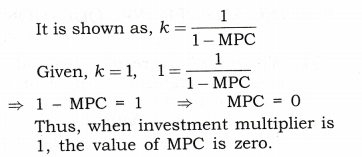Question 5. If the ratio of marginal propensity to consume and marginal propensity to save is 4 : 1, the value of investment multiplier will be 4. [CBSE AI 2010]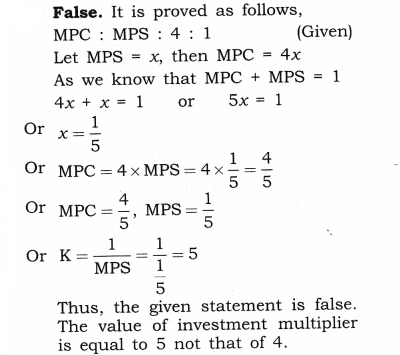V. Long Answer Type Questions (6 Marks)
Question 1. Why must aggregate demand be equal to aggregate supply at the equilibrium level of income and output? Explain with the help of a diagram. Or [CBSE 2006] Explain how is equilibrium level of income and employment established through AD and AS?
Or
Explain determination of equilibrium level of income using consumption plus investment approach. Use diagram. [CBSE 2008]

1.  It refers to the point that has come to be established under the given condition of aggregate demand and aggregate supply, and has tendency to stick to that level under this given condition where Aggregate Demand= Aggregate Supply.
2.  If due to some disturbance, we divert from that position, the economic forces will work in such a manner so as to drive us back to the original position, i.e., aggregate demand is equal to aggregate supply.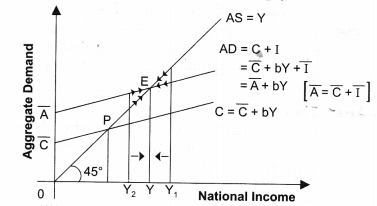3.  In the above mentioned figure, at point P, income = consumption, which is known as to be a break-even point. The equilibrium level of national income is attained at point E, where aggregate demand = aggregate supply.
4.  If due to some disturbance we divert from our position, like when AD > AS [at Y2], then, production will have to be increased to meet the excess demand. Consequently, national income will increase. As we know positive relationship exists between national income and consumption, so consumption will increase, which will thereby increase the aggregate demand till we reach the equilibrium.
5. As against it, when AD < AS [at Y1], then there would be stockpiling and producers will produce less. National income will fall and as a result consumption will start falling, which will thereby reduce the aggregate demand till we reach the equilibrium.

Question 2. Explain the meaning of equilibrium level of income and output using saving and investment approach. Use a diagram.[CBSE 2008, Sample Paper 2010] Or
What changes will take place to bring an economy in equilibrium if (i) planned savings are greater than planned investment, and (ii) planned savings are less than planned investment.
Or [CBSE.2009]
Using the ‘saving and investment’ approach explain how is the equilibrium level of national income determined? Also explain what will happen if the equilibrium condition is not fulfilled.
[Sample Paper 2013] Or
Explain how the economy achieves equilibrium level of income using Savings-Investment (S-I) approach.[CBSE Sample Paper 2016]

1. It refers to the point that has come to be established under the given condition of aggregate demand and aggregate supply, and has tendency to stick to that level under this given condition where Aggregate Demand (AD) = Aggregate Supply (AS).
CONSUMPTION(C) + Investment(I) = CONSUMPTION(C) + Saving(S)
I = S
2.  If due to some disturbance, we divert from that position, the economic forces will work in such a manner so as to drive us back to the original position, i.e., Saving is equal to Investment.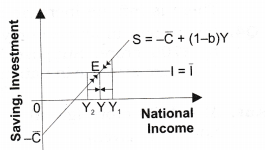3. In the above figure, the equilibrium level of national income is attained at point E, where saving = investment which is derived from a point where S = I.
4. If due to some disturbance we divert from our position like when investment > saving [at Y2], then production will have to be increased to meet the excess demand. Consequently, national income will increase leading to rise in saving until saving becomes equal to investment. It is here that equilibrium level of income is established because what the savers intend to save becomes equal to what the investors intend to invest.
5. As against it, when saving > investment [at Y1], then there would be stockpiling and producers will produce less. National income will fall and as a result saving will start falling until it becomes equal to investment. It is here the equilibrium level of income is derived.

Question 3. Explain the working of investment multiplier with the help of a numerical example. Or [CBSE 2005]
Explain with the help of a numerical example how does increase in investment in an economy affect its level of income? [CBSE AI 2005]
Or
Explain the dynamic multiplier. Or
Explain the income propagation process due to change in investment.
Or
Explain the process of working of the ‘investment multiplier with the help of a numerical example. [CBSE Sample Paper 2014]

Answer: The process of investment multiplier is as under:

1.  It can be illustrated with the help of a simple example. We know that one man’s expenditure is another man’s income.
2.  Suppose, the government of a country spends Rs 100 crore on building roads. National income of the country automatically rises by Rs 100 crore in Round 1.
3. Now suppose MPC is 0.5, people working in the investment industry will spend Rs 50 crore on new consumption goods.
4. The consumer goods industry will have an extra income of Rs 50 crore. Assume the MPC for the whole society is 0.5, people working in these consumer goods industry would again spend 50% of their additional income of Rs 50 crore (which works out to be 25 crore) on more consumer goods.
5. These Rs 25 crore will, thus, become the income for others. This will continue till total increase in income becomes k times the increment of investment.
6.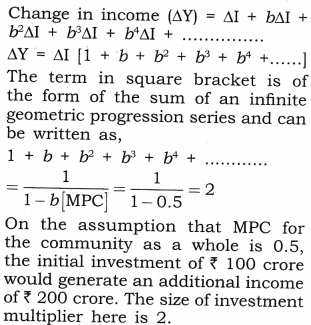VI. Higher Order Thinking Skills
Question 1. In an economy the entire increase in income is saved. What will be the value of multiplier? [1 mark]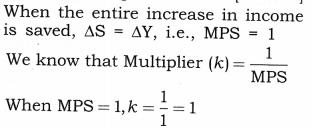Question 2. There is increase in investment of Rs 1000 crore in an economy. MPC is zero. What is the total increase in income? Calculate. [3-4 Marks]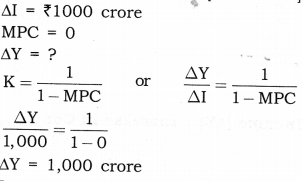Question 3. Investment increases by Rs 500 crores in an economy. MPS is zero. What is the total increase in income? Calculate.[3-4 Marks]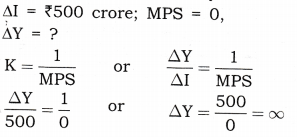There is infinite increase in income.

Question 4. Investment in an economy increases by Rs 700 crore. Suppose MPS is 1. Calculate increase in national income.[3-4 Marks]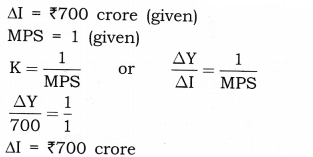Question 5. Derive the multiplier when MPS is:
(i) 0.10 (ii) 0.20 (iii) 0.25.
Using these multiplier values, find the change in the equilibrium level of income that results from Rs 20 crore decrease in investment. [3-4 Marks]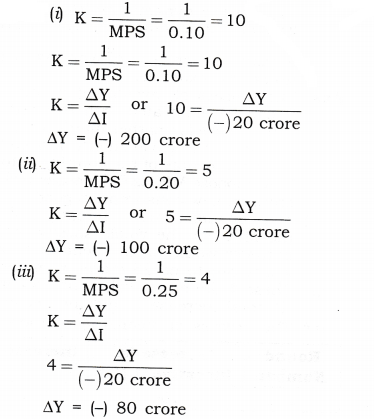Question 6. The savings function of an economy is S = -200 + 0.25 Y. The economy is in equilibrium when income is equal to Rs 2,000. Calculate: [3-4 Marks]
(a) Investment expenditure at equilibrium level of income.
(b) Autonomous consumption.

Answer: S = -200 + 0.25 Y
(a) At equilibrium planned savings are equal to planned investment. Equilibrium level of income is ?2,000. Substituting the value of Y in the savings function, we get;
S = -200 + 0.25 x 2,000 S = 300; I = Rs 300 Thus, investment expenditure at equilibrium level of income is Rs 300.
(b) Consumption + Savings = Income Autonomous consumption means the level of consumption expenditure when income is zero.
When Y = 0, Savings = -200 So, autonomous consumption = Rs 200

Question 7. What are the elements that private investor demand for investment depends? [3-4 Marks]
Answer: A private investor’s demand for investment depends on two things:
(a) The rate of return on investment or M.E.I: The expected rate of return from an additional unit of investment is called marginal efficiency of investment (M.E.I). It is defined as the expected rate of return of an additional unit of capital goods. M.E.I is very important factor in determining the investment demand. M.E.I. is determined by two factors:

1.  Supply Price: The cost of replacing the machine under consideration with a brand new machine is known its supply price. For example, if a machine of Rs 1 lakh is replaced in place of old machine, then Rs 1 lakh is the supply price.
2. Prospective Yields: It refers to expected net returns (of all costs) from the capital asset over its lifetime. For example, if the given machine is expected to yield revenue of Rs 10,000 and running expenditure is Rs 2000, the prospective yield will be, 10000 – 2000 = Rs 8000.
3. Formula of Marginal efficiency of investment: In the given examples, marginal efficiency of investment will be,8000 x 100 = 8%,10000

(b) The Market Rate of Interest: It refers to cost of funds borrowed for financing the investment. There exists inverse relationship between rate of interest and investment demand. Higher interest implies lower level of investment demand.
(c) Decision whether to invest or not The investor goes on making additional investments until M.E.I becomes equal to the rate of interest. If M.E.I is greater than the rate of interest, the investors has to increase the investment and if the rate is higher than the M.E.I, no investment is to be made.
For example, if an entrepreneur has to pay 15% market rate of interest on the loan taken by him and he expected rate of profit i.e., M.E.I. is 30%, then he will surely go for the investment and will continue making investment till M.E.I. = Rate of Interest (ROI).

Question 9. In an economy the autonomous investment is 60 and the marginal propensity to consume is 0.8. If the equilibrium level of income is 400, then the autonomous consumption is 30. True or False? Justify your answer.[CBSE Sample Paper 2014] [3 Marks]
I = 60
C = C¯ + bY = C¯ + 0.8Y
If equilibrium level of income is 400, then C =C¯ + 0.8 x 400 = C¯+ 320
Thus, 60 + C¯+320 = 400
C¯= 20
The given value of autonomous consumption is incorrect. The correct value is 20.

VII.Value Based Question
Question 1. Saving provides economic security in future, but why is it not good from the viewpoint of investment multiplier?[1 Mark]
Answer: Money supply is reduced by increase in savings which creates the situation of deficient demand in economy; consequently it reduces the functioning of investment multiplier.
Value: Analytic.

VIII. Application Based Questions
Question 1. In an economy the equilibrium level of income is Rs 12,000 crore. The ratio of marginal propensity to consume and marginal propensity to save is 3: 1. Calculate the additional investment needed to reach a new equilibrium level of income of Rs 20,000 crore.[CBSE AI 2010][3-4 Marks]